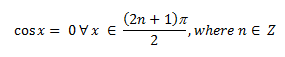# Trigonometric Functions - Domain and Range

All trigonometric functions are basically the trigonometric ratios of any given angle. For example if we take the functions, f(x)=sin x, f(z) = tan z, etc, we are considering these trigonometric ratios as functions. Since they are considered to be functions, they will have some domain and range. In the upcoming discussion, we shall figure out the domain and range of trigonometric functions.

## Domain and Range of Trigonometric Functions (Sin, Cos, Tan)

To begin with, let us consider the simplest trigonometric identity:

sin2x + cos2 x = 1

From the given identity, the following things can be interpreted:

cos2x = 1- sin2 x

cos x = √(1- sin2x)

Now we know that cosine function is defined for real values therefore the value inside the root is always non-negative. Therefore,

1- sin2x ≥ 0

sin2x ≤ 1

sin x ∈ [-1, 1]

Hence, we got the range and domain for sine function.

Similarly, following the same methodology,

1- cos2x ≥ 0

cos2x ≤1

cos x ∈ [-1,1]

Hence, for the trigonometric functions f(x)= sin x and f(x)= cos x, the domain will consist of the entire set of real numbers, as they are defined for all the real numbers. The range of f(x) = sin x and f(x)= cos x will lie from -1 to 1, including both -1 and +1, i.e.

• -1 ≤ sin x ≤1
• -1 ≤ cos x ≤1

Now, let us discuss the function f(x)= tan x. We know, tan x = sin x / cos x. It means that tan x will be defined for all values except the values that will make cos x = 0, because a fraction with denominator 0 is not defined. Now, we know that cos x is zero for the angles  π/2, 3 π/2, 5 π/2 etc. therefore,Hence, for these values, tan x is not defined.

$$\begin{array}{l}\text{So, the domain of f(x) = tan x will be } R – \frac{(2n+1)π}{2} \text{ and the range will be set of all real numbers, R.}\end{array}$$

### Domain and Range for Sec, Cosec and Cot Functions

We know that sec x, cosec x and cot x are the reciprocal of cos x, sin x and tan x respectively. Thus,

sec x = 1/cos x

cosec x = 1/sin x

cot x = 1/tan x

Hence, these ratios will not be defined for the following:

1. sec x will not be defined at the points where cos x is 0. Hence, the domain of sec x will be R-(2n+1)π/2, where n∈I. The range of sec x will be R- (-1,1). Since, cos x lies between -1 to1, so sec x can never lie between that region.
2. cosec x will not be defined at the points where sin x is 0. Hence, the domain of cosec x will be R-nπ, where n∈I. The range of cosec x will be R- (-1,1). Since, sin x lies between -1 to1, so cosec x can never lie in the region of -1 and 1.
3. cot x will not be defined at the points where tan x is 0. Hence, the domain of cot x will be R-nπ, where n∈I. The range of cot x will be the set of all real numbers, R.

### Video Lesson on Trigonometry